# How to Get the Path of Current Worksheet in Excel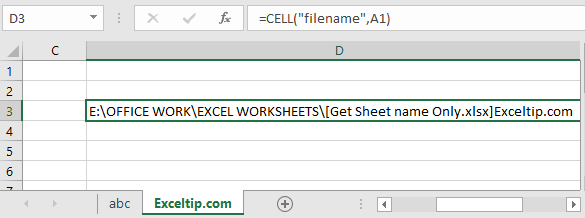In this article, we will learn How to Get the Path of Current Worksheet in Excel.

CELL function in Excel

Cell function in Excel gets you the information regarding worksheets like col, contents, filename, ..etc.

Syntax

 =CELL("filename",A1)

“filename” : gets the full name of the sheet of the reference cell

A1 : Sheet’s  cell referenceBut we need to extract just the sheet name. Basically the last name.

As you can see the sheet name starts after ] (closed big bracket sign). For that we just needs its position in the text and then we will extract the sheet name.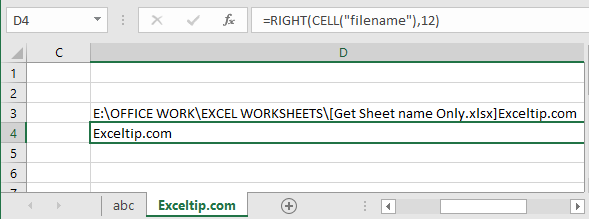Here I counted the characters from the last of the result which was 12. But we cannot do this every time.

So for extracting the sheet name from the full name, we need to find the position of the ] (closed big bracket sign)

Use the formula to find ]

 =FIND("]",CELL("filename",A1))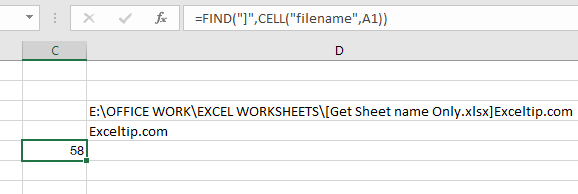58 is the position of ] .

Now we our formula is completed now

 =MID(CELL("filename",A1),FIND("]",CELL("filename",A1))+1,255)

Explanation:

CELL("filename",A1) : Gets you the full name of the worksheet

FIND("]",CELL("filename",A1))+1 : this function gets you the position of ] and add +1 because we need the position of first char of only sheet name.

255 : Max word limit of sheet name by Excel.

MID : MID function extracts the specific substring from the text using the position from start to end.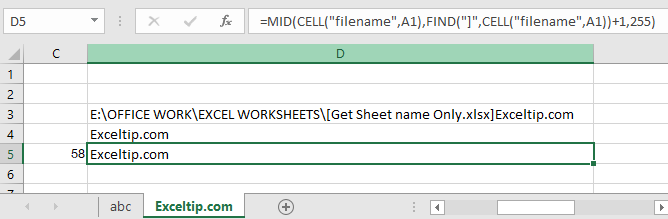Here we extracted the filename using the formula.

Now we have one more formula, instead of using MID function. Use the RIGHT function.

RIGHT function extracts the specific substring from the last of the text.

Use the formula:

 =RIGHT(CELL("filename",A2),LEN(CELL("filename",A2))-FIND("]", CELL("filename",A2)))

Explanation:

FIND("]", CELL("filename",A2)) : Extracts the position of sheet name char.

LEN(CELL("filename",A2)) : gets the length of the text(filename)

RIGHT : RIGHT function extracts the string from the text using its position.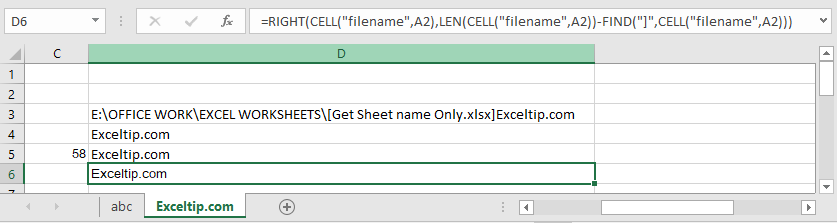As you can see, this function works fine too. Yesss...Now we have 2 different formula with logic used to extract only the sheet name.

Get only the sheet name using VBA in Excel

VBA example

We have data in three sheets and we want to consolidate data from all of them to the main sheet. Data from each sheet should have sheet name at the end.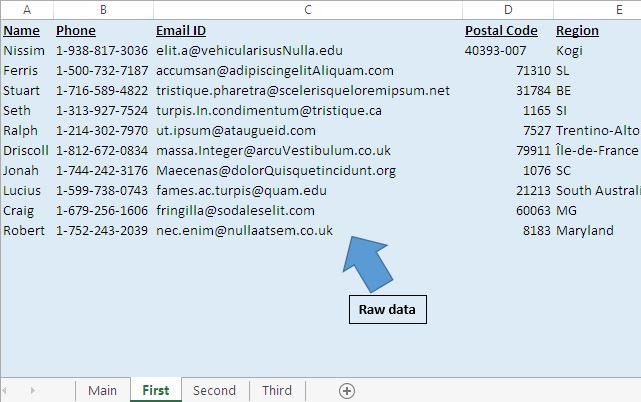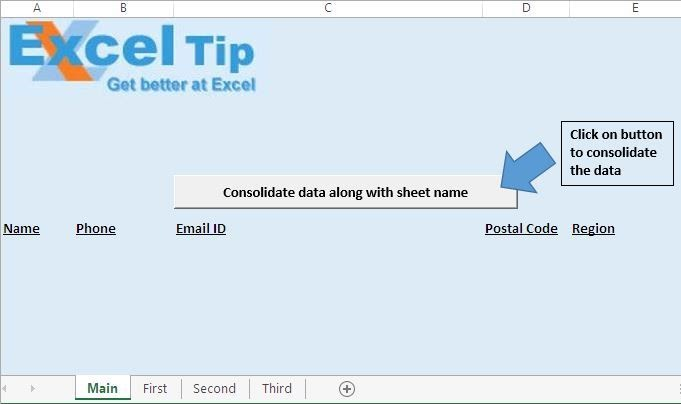On clicking “Consolidate data along with sheet name” button, data will be consolidated on the main sheet.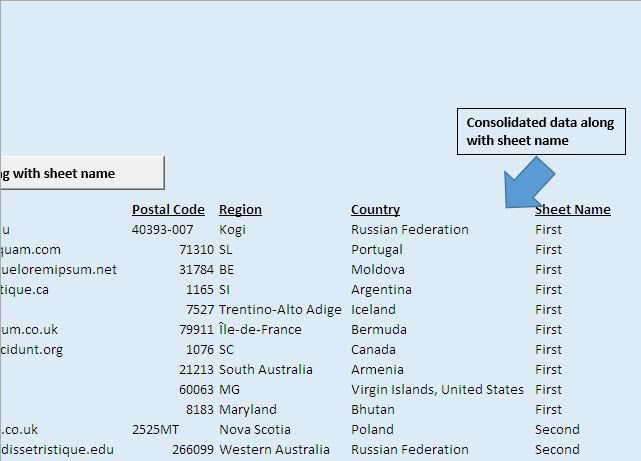Code explanation

```SheetCount = Application.Worksheets.Count
The above code is used to get the count of worksheet within the workbook.
LastRow = ActiveCell.SpecialCells(xlLastCell).Row
The above code is used to get the row number of the last cell.
Range("A2:F" & LastRow).Select
The above code is used to select all the data starting from cell A2.
Selection.Copy
The above code is used to copy the selected data.
Range(Selection, Cells(LastRow, 7)).Value = Sheets(Counter).Name
The above code is used to assign the sheet name to the selected cell.

```

```Option Explicit

Sub ConsolidateDataWithSheetName()

'Declaring variables
Dim Counter As Integer
Dim SheetCount As Integer
Dim LastRow As Long

Application.ScreenUpdating = False

'Getting the count of worksheets in the workbook
SheetCount = Application.Worksheets.Count

For Counter = 2 To SheetCount

Sheets(Counter).Activate

Range("A2").Select

'Getting the row number of the last cell
LastRow = ActiveCell.SpecialCells(xlLastCell).Row

'Selecting all the data in the workbook
Range("A2:F" & LastRow).Select

'Copying the selected data
Selection.Copy

Sheets("Main").Activate

Range("A2").Select
LastRow = ActiveCell.SpecialCells(xlLastCell).Row
LastRow = LastRow + 1

'Pasting the copied data to last row on the Main sheet
Cells(LastRow, 1).Select
ActiveSheet.Paste

Cells(LastRow, 7).Select
LastRow = ActiveCell.SpecialCells(xlLastCell).Row

'Adding the sheet name along with the data
Range(Selection, Cells(LastRow, 7)).Value = Sheets(Counter).Name

Next

End Sub

```

Hope this article about How to Get the Path of Current Worksheet in Excel is explanatory. Find more articles on calculating values and related Excel formulas here. If you liked our blogs, share it with your friends on Facebook. And also you can follow us on Twitter and Facebook. We would love to hear from you, do let us know how we can improve, complement or innovate our work and make it better for you. Write to us at info@exceltip.com.

Related Articles :

All About Excel Named Ranges : excel ranges that are tagged with names are easy to use in excel formulas. Learn all about it here.

The Name Box in Excel : Excel Name Box is nothing but a small display area on top left of excel sheet that shows the name of active cell or ranges in excel. You can rename a cell or array for references.

How to Get Sheet name of worksheet in Excel : CELL Function in Excel gets you the information regarding any worksheet like col, contents, filename, ..etc.

How to use Dynamic Named Ranges in Excel : A dynamic name range is a name used for the array or range that expands and shrinks according to data. Learn more about dynamic named ranges here.

How to Create Drop Down List with Color in Excel : Add colors to the drop down list in Excel using Conditional formatting and data validation.

Join first and last name in excel : Join two or more values or names using the CONCATENATE function or & operator. This article explains how to add two or more cells in Excel.

Popular Articles :

How to use the IF Function in Excel : The IF statement in Excel checks the condition and returns a specific value if the condition is TRUE or returns another specific value if FALSE.

How to use the VLOOKUP Function in Excel : This is one of the most used and popular functions of excel that is used to lookup value from different ranges and sheets.

How to Use SUMIF Function in Excel : This is another dashboard essential function. This helps you sum up values on specific conditions.

How to use the COUNTIF Function in Excel : Count values with conditions using this amazing function. You don't need to filter your data to count specific values. Countif function is essential to prepare your dashboard.

Terms and Conditions of use

The applications/code on this site are distributed as is and without warranties or liability. In no event shall the owner of the copyrights, or the authors of the applications/code be liable for any loss of profit, any problems or any damage resulting from the use or evaluation of the applications/code.